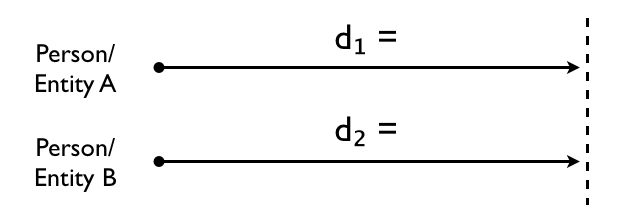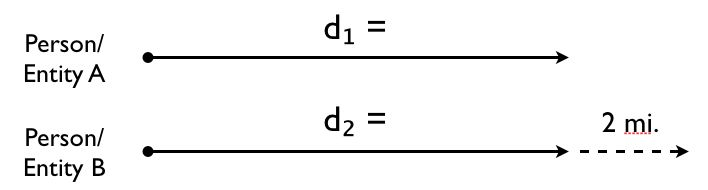Learn the 4 Things You Must Know Before Taking the GMAT!   Download it Now!

# Motion Problems: “Catch Up”

Motion problems are among the most common word problems you’ll encounter on the Quantitative section of the GMAT. These problem solving questions fall into one of three categories:

1. Motion in Opposite Directions
2. Motion in the Same Direction (“Catch Up” Problems)
3. Round Trip Questions

In this lesson we’ll cover Motion in the Same Direction.

### 2. Motion in the Same Direction (“Catch Up”): The Basics

All types of motion questions on the GMAT require you to apply the following basic Distance Formula:

Distance = Rate * Time

The general idea with Same Direction — or “Catch Up” — motion questions is that you have two entities moving in the same direction at different rates of speed. One entity usually starts after the other entity and travels at a faster rate of speed to “catch up” with the first entity. The question itself will generally ask you to figure out how long it takes for the second entity to catch up with the first entity, or perhaps how much distance the second entity must travel before overtaking (i.e. “catching up with”) the first entity.

Regardless of what information is given to you and what you’re ultimately asked to solve for, these questions always come down to solving for one of the variables in the Distance Formula. There are three crucial steps to finding the missing piece that the question is asking you to solve for.

### The Strategy

Step 1: Draw a diagram illustrating what the problem is telling you. This will help you visualize what you’re solving for and get your mind around the question itself.

The diagram will usually look something like this:Step 2: The best starting point for solving Same Direction questions is to use this formula:

d = d₂

Step 3: Set up an individual distance formula for each entity, d₁ and d₂, and set them equal to each other.* You may be asked to find the distance traveled by each entity, or perhaps you’ll simply be asked how long (i.e. time in the Distance Formula) it takes one entity to catch the other.

* Note: On harder questions, Person/Entity 2 may start from further away or overtake Person/Entity 2 by a certain distance. In that case, obviously d₁ and d₂ will not be equal. You’ll want to use your diagram as a guide and adjust your equation as necessary. For example, if the problem asks you how long it will take for Person/Entity 2 to catch and overtake Person/Entity 1 by 2 miles, then your diagram may look like this:Thus, for those two distances to be “equal,” you’ll have to adjust your equation as follows (assuming the rate is given in miles):

d + 2 = d₂

In other words, Person/Entity 1 would in theory have to travel an additional 2 miles in order for his distance to equal Person/Entity 2’s distance.

### On the GMAT

This will all make more sense once you see how this strategy is applied to a sample GMAT question. Watch this video for a step-by-step application: